﻿ 一起来写个简单的解释器（10） – 魔力Python

# 一起来写个简单的解释器（10）

Python教程 4300浏览

```PROGRAM Part10;
VAR
number     : INTEGER;
a, b, c, x : INTEGER;
y          : REAL;

BEGIN {Part10}
BEGIN
number := 2;
a := number;
b := 10 * a + 10 * number DIV 6;
c := a - - b
END;
x := 11;
y := 20 / 7 + 3.14;
{ writeln('a = ', a); }
{ writeln('b = ', b); }
{ writeln('c = ', c); }
{ writeln('number = ', number); }
{ writeln('x = ', x); }
{ writeln('y = ', y); }
END.  {Part10}```

• 程序头
• 变量声明
• DIV关键字
• 注释

• 如何解析和解释Pascal程序头；
• 如何解析Pascal的变量声明；
• 如何让解释器支持DIV关键字的整数除法和斜杠“/”的浮点数除法；
• 如何增加对注释的支持。

1、Program

program : PROGRAM variable SEMI block DOT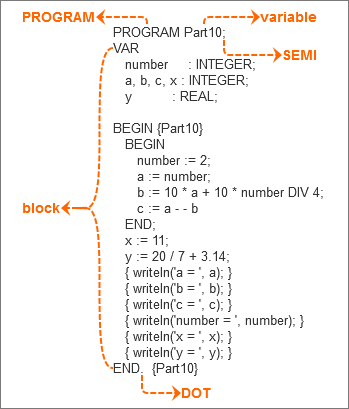2、block

block : declarations compound_statement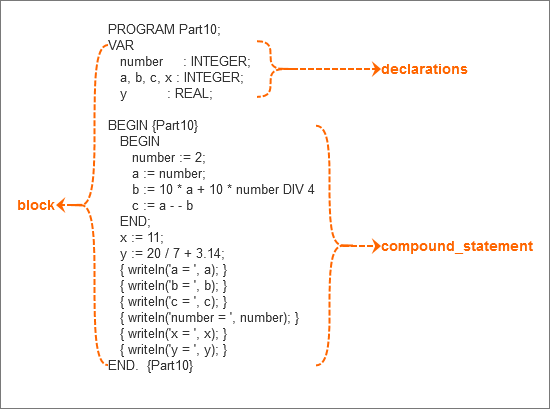3、declarations

declarations : VAR (variable_declaration SEMI)+ | empty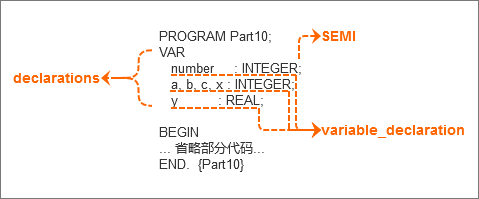4、variable_declaration

variable_declaration : ID (COMMA ID)* COLON type_spec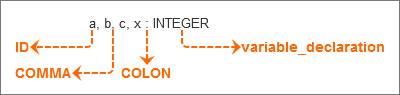5、type_spec

type_spec : INTEGER

6、没有变化的文法

compound_statement : BEGIN statement_list END

statement_list : statement | statement SEMI statement_list

statement : compound_statement | assignment_statement | empty

assignment_statement : variable ASSIGN expr

empty :

expr : term ((PLUS | MINUS) term)*

7、term

term : factor ((MUL | INTEGER_DIV | FLOAT_DIV) factor)*

15 / 6 = 2.5

15 DIV 6 = 2

8、factor

factor : PLUS factor | MINUS factor | INTEGER_CONST | REAL_CONST | LPAREN expr RPAREN | variable

• 更新词法分析器（Lexer）
• 更新语法分析器（Parser）
• 更新解释器（Interpreter）

• 实数类型：REAL（之前的整数INTEGER作为整数类型，无需再添加）
• 整数：INTEGER_CONST
• 实数：REAL_CONST
• 整数除法符号：INTEGER_DIV
• 浮点数除法符号：FLOAT_DIV
• 程序标记：PROGRAM
• 变量声明标记：VAR
• 逗号：COMMA
• 冒号：COLON

```INTEGER = 'INTEGER'  # 整数类型
REAL = 'REAL'  # 实数类型
INTEGER_CONST = 'INTEGER_CONST'  # 整数（因子）
REAL_CONST = 'REAL_CONST'  # 实数（因子）
PLUS = 'PLUS'  # 加
MINUS = 'MINUS'  # 减
MUL = 'MUL'  # 乘
INTEGER_DIV = 'INTEGER_DIV'  # 整数除法
FLOAT_DIV = 'FLOAT_DIV'  # 浮点数除法
LPAREN = 'LPAREN'  # 左括号
RPAREN = 'RPAREN'  # 右括号
ID = 'ID'  # 变量名称
ASSIGN = 'ASSIGN'  # 赋值符号
BEGIN = 'BEGIN'  # 开始标记
END = 'END'  # 结束标记
SEMI = 'SEMI'  # 分号
DOT = 'DOT'  # 点（程序结束符）
PROGRAM = 'PROGRAM'  # 程序
VAR = 'VAR'  # 变量声明标记
COLON = 'COLON'  # 冒号
COMMA = 'COMMA'  # 逗号
EOF = 'EOF'  # 结束符号```

• VAR：变量声明
• DIV：整数除法
• PROGRAM：程序
• 整数类型：INTEGER
• 实数类型：REAL

```RESERVED_KEYWORDS = {  # 保留字
'PROGRAM': Token('PROGRAM', 'PROGRAM'),
'VAR': Token('VAR', 'VAR'),
'DIV': Token('INTEGER_DIV', 'DIV'),
'INTEGER': Token('INTEGER', 'INTEGER'),
'REAL': Token('REAL', 'REAL'),
'BEGIN': Token('BEGIN', 'BEGIN'),
'END': Token('END', 'END'),
}```

1、更新词法分析器（Lexer）

```def skip_comment(self):  # 添加跳过注释内容到的方法
while self.current_char != '}':  # 如果当前字符不是注释结束符号

```def number(self):  # 添加处理整数和实数的方法
result = ''  # 定义保存数字字符串的变量
while self.current_char is not None and self.current_char.isdigit():  # 如果当前字符不是None并且为整数
result += self.current_char  # 连接已有数字字符串
if self.current_char == '.':  # 如果当前字符为小数点
result += self.current_char  # 连接已有数字字符串
while self.current_char is not None and self.current_char.isdigit():  # 如果当前字符不是None并且为整数（小数点后均为整数）
result += self.current_char  # 连接已有数字字符串

```def get_next_token(self):
while self.current_char is not None:
if self.current_char == '{':  # 如果当前字符为注释起始标记
self.skip_comment()  # 跳过注释内容
continue  # 继续获取下一记号
...省略部分代码...
if self.current_char.isdigit():  # 当前字符为整数时
return self.number()  # 获取整数或实数记号
if self.current_char == ':':  # 当前字符为冒号时
if self.current_char == ',':  # 当前字符为逗号时
...省略部分代码...
if self.current_char == '/':  # 当前字符为斜杠时
...省略部分代码...

2、更新语法分析器（Parser）

```class Program(AST):  # 定义程序节点
def __init__(self, name, block):  # 程序由名称和语句块组成
self.name = name
self.block = block

class Block(AST):  # 定义语句块节点
def __init__(self, declarations, compound_statement):  # 语句块由声明和符合语句组成
self.declarations = declarations
self.compound_statement = compound_statement

class VarDecl(AST):  # 定义变量声明节点
def __init__(self, var_node, type_node):  # 变量声明由变量和类型组成
self.var_node = var_node
self.type_node = type_node

class Type(AST):  # 定义类型节点
def __init__(self, token):
self.token = token
self.name = token.value```

```def block(self):  # 构造块节点的方法
declarations = self.declarations()
compound_statement = self.compound_statement()
node = Block(declarations, compound_statement)  # 块节点由声明节点和符合语句节点组成
return node

def declarations(self):  # 构造声明节点的方法
declarations = []  # 声明节点包含多个变量声明节点
if self.current_token.value_type == VAR:  # 如果当前记号为变量
self.eat(VAR)  # 验证记号
while self.current_token.value_type == ID:  # 遍历变量名称
declarations.extend(self.variable_declaration())  # 声明列表中添加变量声明
self.eat(SEMI)  # 验证分号
return declarations  # 返回声明节点列表

def variable_declaration(self):  # 构造变量声明节点的方法
var_nodes = [Variable(self.current_token)]  # 第一个变量声明节点添加到变量声明节点列表
self.eat(ID)  # 验证变量名称记号
while self.current_token.value_type == COMMA:  # 遍历逗号
self.eat(COMMA)  # 验证逗号
var_nodes.append(Variable(self.current_token))  # 添加变量节点到变量节点列表
self.eat(ID)  # 验证变量名称记号
self.eat(COLON)  # 验证冒号
type_node = self.type_spec()  # 一组变量声明的类型节点
var_declarations = [VarDecl(var_node, type_node) for var_node in var_nodes]  # 生成变量声明列表
return var_declarations  # 返回变量声明节点列表

def type_spec(self):  # 构造变量类型节点的方法
token = self.current_token  # 获取当前记号
if token.value_type == INTEGER:  # 如果是整数类型
self.eat(INTEGER)  # 验证整数记号
else:  # 否则
self.eat(REAL)  # 验证实数记号
node = Type(token)  # 创建类型节点
return node  # 返回类型节点```

```def program(self):
self.eat(PROGRAM)  # 验证程序开始标记
var_node = self.variable()  # 获取变量节点
program_name = var_node.name  # 获取程序名称
self.eat(SEMI)  # 验证分号
block_node = self.block()  # 获取块节点
node = Program(program_name, block_node)  # 创建程序节点
self.eat(DOT)  # 验证程序结束符号
return node  # 返回程序节点

def term(self):
node = self.factor()
while self.current_token.value_type in (MUL, INTEGER_DIV, FLOAT_DIV):  # 除法修改为整数除法和浮点数除法
token = self.current_token
if token.value_type == MUL:
self.eat(MUL)
elif token.value_type == INTEGER_DIV:  # 整数除法
self.eat(INTEGER_DIV)
elif token.value_type == FLOAT_DIV:  # 浮点数除法
self.eat(FLOAT_DIV)
node = BinaryOperator(node, token, self.factor())
return node

def factor(self):
current_token = self.current_token

if current_token.value_type == PLUS:
...省略部分代码...
elif current_token.value_type == INTEGER_CONST:  # 整数
self.eat(INTEGER_CONST)
return Num(current_token)
elif current_token.value_type == REAL_CONST:  # 实数
self.eat(REAL_CONST)
return Num(current_token)
elif current_token.value_type == LPAREN:
...省略部分代码...```

```PROGRAM Part10AST;
VAR
a, b : INTEGER;
y    : REAL;

BEGIN {Part10AST}
a := 2;
b := 10 * a + 10 * a DIV 4;
y := 20 / 7 + 3.14;
END.  {Part10AST}```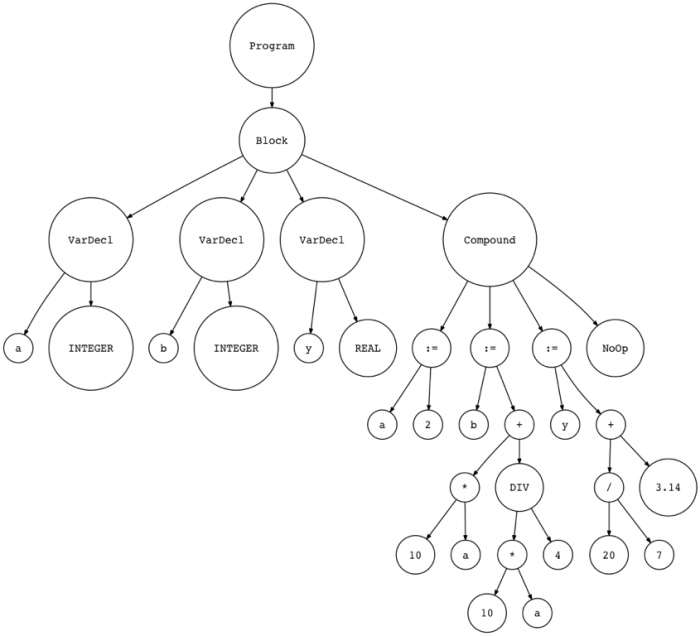3、更新解释器（Interpreter）

• visit_Program
• visit_Block
• visit_VarDecl
• visit_Type

```def visit_Program(self, node):  # 添加访问程序的方法
self.visit(node.block)  # 访问语句块

def visit_Block(self, node):  # 添加访问语句块的方法
for declaration in node.declarations:  # 遍历声明列表
self.visit(declaration)  # 访问声明
self.visit(node.compound_statement)  # 访问复合语句

def visit_VarDecl(self, node):  # 添加访问变量声明的方法
pass  # 无需处理

def visit_Type(self, node):  # 添加访问类型的方法
pass  # 无需处理```

```def visit_BinaryOperator(self, node):
if node.operator.value_type == PLUS:
return self.visit(node.left) + self.visit(node.right)
elif node.operator.value_type == MINUS:
return self.visit(node.left) - self.visit(node.right)
elif node.operator.value_type == MUL:
return self.visit(node.left) * self.visit(node.right)
elif node.operator.value_type == INTEGER_DIV:  # 如果是整数除法
return self.visit(node.left) // self.visit(node.right)  # 返回整数除法运算结果
elif node.operator.value_type == FLOAT_DIV:  # 如果是浮点数除法
return self.visit(node.left) / self.visit(node.right)  # 返回浮点数除法运算结果```

• 文法中添加了新规则并更新了现有规则；
• 将新的记号和相关方法添加到词法分析器中，并更新和修改了现有的方法；
• 将新的AST节点类添加到语法分析器中，以构建新的语言结构；
• 将新的文法规则对应的新方法添加到了语法分析器中，并更新现有的方法；
• 将新的访问方法添加到了解释器中，并更新现有访问方法。

• 解释器现在可以处理程序头；
• 变量现在可以使用VAR关键字来声明；
• DIV关键字用于整数除法，斜杠“/”用于浮点数除法。